# Modeling the Earth and Sun (2 body orbits) using general relativity?

• I
James1238765
@Ibix from differential equations perspective, could we recover completely ##g_{ij}## from the full set of descendant ##\Gamma^i_{jk}## (post 33)?

As to why I insist on trying this, even if the solution is wrong, the practice is often invaluable. We can see in a single picture if the ##T_{ij}## solution is wrong or right.

2022 Award
No idea.

James1238765
Adding 2 sources for ##g_{ij}## could be done pointwise as follows:

$$g_{total} (r, \theta, \phi) = g_{ij} (r, \theta, \phi) +$$
$$g_{ij}(Sph_r(Cart_x(r,\theta,\phi)+dist_x, Cart_y(r,\theta,\phi)+dist_y, Cart_z(r,\theta,\phi)+dist_z))$$

Single source metric is a vacuum solution:Two sources at cartesian distance 50,000 is a mess (the further the messier):Mentor
@James1238765 I have no idea what anything in your post #38 means.

•vanhees71
James1238765
Sorry the coordinate notation was abstract. Here's a real example, the point (1, 1, 1) in spherical coordinate is first transformed into cartesian via:

$$x = r \sin \theta \ cos \phi$$
$$y = r \sin \theta \ sin \phi$$
$$z = r \ cos \theta$$

we obtain the new cartesian coordinates: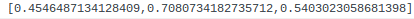Next we add some cartesian offset we like, eg. 10 to each coordinate: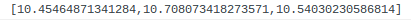Then we transform back into spherical coordinates via:

$$r = \sqrt {x^2 + y^2 + z^2}$$
$$\theta = \arccos \frac{z}{r}$$
$$\phi = sign(y) \arccos \frac{x}{\sqrt{x^2 + y^2}}$$

we obtain the new spherical coordinates: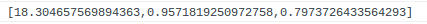At each gridpoint, ##g_{ij}## is defined in terms of every ##\{r, \theta, \phi\}## values. By adding pointwise the ##g_{ij}## values for both (1,1,1) and (18.3, 0.95, 0.79) we sum as if two different sources of ##g## are located at 10 distance apart cartesian-ly.

Mentor
Next we add some cartesian offset
The offset will be time dependent.

Then we transform back into spherical coordinates
I'm not sure why you would do this. For numerical simulations Cartesian coordinates actually work better.

James1238765
The heavyweight computations used original Schwarzchild coordinates, and I was wary if changing to Cartesian suddenly might break something. Yes otffset being time dependant would mean moving bodies, though the solution as currently obtained gives nonsensical ##T_{ij}## anyways, so it's still some nice idea to dream about for now...

Mentor
The heavyweight computations used original Schwarzchild coordinates
Which computations are you referring to?

James1238765
all that really long chain beginning with ##g_{ij} \rightarrow g^{ij} \rightarrow \Gamma^i_{jk} \rightarrow R^i_{jkl} \rightarrow R_{ij} \rightarrow R##

Mentor
the solution as currently obtained gives nonsensical ##T_{ij}## anyways
That's because you need to specify ##T_{ij}## first (to be zero outside the two bodies, and with some reasonable ansatz for their interiors), at least at some initial instant of time. @Ibix mentioned the ADM formalism in a previous post; for the kind of simulation you are considering that is the best technique I'm aware of. Basically you specify initial conditions on some spacelike slice in terms of an initial stress-energy distribution, solve constraint equations (which are one piece of the Einstein Field Equations) to obtain the metric on the spacelike slice, and then evolve things forward in time using evolution equations (the remaining piece of the Einstein Field Equations).

James1238765
Ok. will try it backward from ##T_{ij}## next.

Are all the reverse algorithms ##R \rightarrow R_{ij} \rightarrow R^i_{jk} \rightarrow \Gamma^i_{jk} \rightarrow g^{ij} \rightarrow g_{ij}## well defined and calculable for all the intermediate quantities?

2022 Award
Ok. will try it backward from ##T_{ij}## next.

Are all the reverse algorithms ##R \rightarrow R_{ij} \rightarrow R^i_{jk} \rightarrow \Gamma^i_{jk} \rightarrow g^{ij} \rightarrow g_{ij}## well defined and calculable for all the intermediate quantities?
See post #7.

Gold Member
2022 Award
@vanhees71 I find that it's hard to always discuss physics conceptually here. What I meant was, what happens when two stars are close to one another is that they will pull each other closer over time.
I find it very hard to discuss physics conceptually, when people ignore the concepts. SCNR.

Go a step back and look at the Newtonian case first. In Newtonian physics space is completely independend of anything physically happen. That's Newon's absolute space. As you well know that doesn't imply that nothing happens at all but we nicely describe all kinds of motions of bodies within Newtonian mechanics, and indeed you can solve the two-body Kepler problem (two point particles being interacting via the Newtonian gravitational force) exactly. The answer is that both bodies run on ellipses around their common center of mass, which is in one focus of each of these two ellipses.

In GR you cannot solve this problem exactly anymore, but for the bodies in our solar system you can (in both the Newtonian calculation and in GR) approximate the description by assuming that the Sun is at rest, because it's so much heavier than any planet in our solar system. Then the GR (approximate) solution is as follows

(a) solve for the Einstein-Hilbert field equations for a spherically symmetric body outside of this body. The result is that you necessarily have a static spacetime given by the Schwarzschild solution.

(b) describe the motion of the planet around the Sun as the motion of a "test particle" in this given fixed static spacetime. You get something very close to a Kepler ellipse with the Sun in one of its foci. The most famous GR effect is that you don't have an exact ellipse but that the perihelion is very slowly rotating in the same sense as the planet moves on its orbit. For mercury this GR perihelion shift is about 43'' per century.

(c) if you now are very accurate then you consider that there's also gravitational-wave radiation due to the motion of the planet around the Sun, and thus the planet looses energy, which implies that the orbit gets smaller with time. For the case of a planet aroudn the Sun that's however unobservable, because it's very tiny.

It can, however, be observed by "pulsar timing", i.e., observing a binary-star system orbiting around each other. In fact the predicted energy loss due to emission of gravitational waves was observed in this way, providing the first (indirect) evidence for the existence of gravitational waves:

https://en.wikipedia.org/wiki/Hulse–Taylor_binary

Note hat in this case you have to take into account the motion as a two-body problem, which cannot be exactly solved within GR. So you have to use approximations, and here a Newtonian approximation with systematic relativistic corrections (in the spirit of the socalled post-Newtonian parametric formalism) is necessary.

Today pulsar timing is among the most accurate tests of general relativity, confirming the GR values for the post-Newtonian parameters at pretty high order with high accuracy.

But nothing moves in the Schwarzchild metric. Inserting a ##t## velocity with ##\Delta s##, the test particle will move, but the bodies generating the curvature itself will not move. Why is this? Because the metric is not dependent on time. A more enlightened metric that changes the ##\Gamma## values over time might be able to encode this gravitational pull between two objects.
I hope the above has made clear that in static spacetimes such as Newtonian spacetime, SR spacetime, or the Schwarzschild spacetime of GR, of course you can describe the motion of objects, and these objects are not doomed to be frozen forever at rest. How do you come to such an idea although in classical mechanics you are taught from the high-school level on how to calculate the motion of bodies under the influence of all kinds of forces?
But because the solutions we have such as Schwarzchild are not ##t## dependent, this is why the picture is frozen. Thus we need to resort to other methods like Newton's gravity to "update" the positions outside of GR. One may endlessly discuss conceptually whether time is really a dimension or a consecutive rendering of frames, but I would rather calculate and not go into this.
No. As I tried to explain above, you can exactly solve the motion of test bodies (or even massless particles describing the propagation of light in the geometrical-optics approximation) in the given Schwarzschild spacetime. These calculations provided the first predictions for tests of GR:

-perihelion shift of mercury: besides the mach larger perihelion shifts due to the disturbance by other heavenly bodies and the deviation of the gravitational field of the Sun from an exactly spherically symmetric one, which were all well understood already in the 19th century and it was known for a long time that the said 43'' per century perihelion shift could not be explained, and it was finally explained by GR as demonstrated by Einstein in 1915/1916 in his first papers about the final version of GR.

-deflection of light by the gravitational field of the Sun: With the final version of GR Einstein got twice the value than you get from a naive Newtonian approximation, which he had used some years before, and this was famously tested in two expeditions to the solar eclipse of 1919 by English astronomers (among them Eddington), and the confirmation of the value according to the final version of GR in 1916 made Einstein the first "shooting star of science" in 1919.

-Shapiro delay: that's a small delay in light propagation due to GR effects. It was predicted by Shapiro in 1964 and first measured by radar reflection on the Venus in 1966/67:

https://en.wikipedia.org/wiki/Shapiro_time_delay

-gravitational red shift of the light coming from the Sun: this was also a prediction by Einstein, and the famous Einstein tower in Potsdam built for the purpose to measuring it, but this was not possible with the technology at this time. It was finally achieved in the early 60ies on the Sun and about 10 years earlier on Serius A and B:

https://en.wikipedia.org/wiki/Gravitational_redshift#Experimental_verification

•James1238765
Mentor
reverse algorithms
What reverse algorithms are you talking about?

James1238765
Starting with ##T_{ij}## we have 16 numbers for each 3D gridpoint, with particular places we want to have all 0 values. The problem seems to be trying to reverse engineer what R and ##R_{ij}## will give rise to this result, then reverse engineer the ##\Gamma^i_{jk}## and all the way to ##g_{ij}## if necessary, to ensure this desired result?

Both R and the 16 ##R_{ij}## numbers must be zero at precisely these same locations we want ##T_{ij} = 0##. How about the 256 ##R^i_{jkl}## numbers, what algorithm will reconstruct these numbers so that the downstream results are as desired?

-------------------------------------------------------

Generalizing to Kerr ##g_{ij}##, we switch to:

$$g_{ij} = \begin{bmatrix} (1 - \frac{2mr}{(r^2+a^2\cos^2\theta)^2})c^2 & 0 & 0 & \frac{2mcra\sin^2\theta}{(r^2+a^2\cos^2\theta)^2} \\ 0 & \frac{(r^2+a^2\cos^2\theta)^2}{r^2+a^2-2mr} & 0 & 0 \\ 0 & 0 & -(r^2+a^2\cos^2\theta)^2 & 0 \\ \frac{2mcra\sin^2\theta}{(r^2+a^2\cos^2\theta)^2)} & 0 & 0 & (r^2+a^2)\sin^2\theta + \frac{2mra^2\sin^4\theta}{(r^2+a^2\sin^2\theta)^2} \end{bmatrix}$$

When ##a=0##, this reduces to Schwarzchild ##g_{ij}##.

The metric for twin sources can be reasonably approximated using a single metric, when the separation distance is very small. The analytic version is called Kerr-rotator [here].

Kerr-Kerr ##T_{ij}## distance 0:Kerr-Kerr ##T_{ij}## distance ##\pm 1##:Kerr-Kerr ##T_{ij}## distance ##\pm 5##: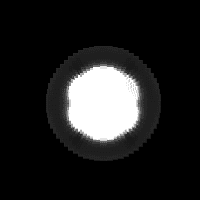Kerr-Kerr ##T_{ij}## distance ##\pm 10##: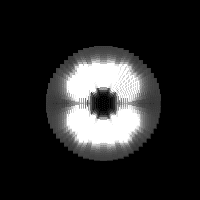Kerr-Kerr ##T_{ij}## distance ##\pm 20##: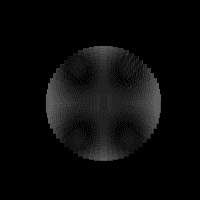Kerr-Kerr ##T_{ij}## distance ##\pm 30##: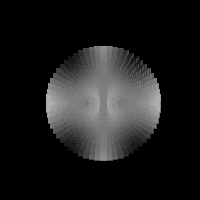Kerr-Kerr ##T_{ij}## distance ##\pm 40##: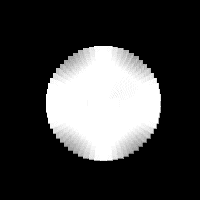Mentor
Starting with ##T_{ij}## we have 16 numbers for each 3D gridpoint
Huh? The stress-energy tensor is symmetric, so it only has 10 independent components, and spacetime is 4D, not 3D.

The problem seems to be trying to reverse engineer what R and ##R_{ij}## will give rise to this result
You don't "reverse engineer" anything. You write down the components of the Einstein Field Equation and solve them. But in a case like this, as has already been noted, you can't solve them analytically, you can only solve them numerically, and to write them down you need an ansatz for the metric in terms of functions of the coordinates.

Since you have isolated regions where the SET is nonzero, with the rest of the spacetime having the SET zero, you actually have to do three solutions, and then join them at boundaries.

The short version: this is a lot more complicated than you seem to imagine, and you would be much better served by trying to look at worked examples from the numerical relativity community, than by trying to guess for yourself.

James1238765
Which Einstein field equation? The geodesic ones will just calculate trajectories, don't they?

What field differential equations precisely will arise from

$$R_{ij} + Rg_{ij} = T_{ij}$$

? I would greatly appreciate it if someone could link to a straightforward worked example in numerical relativity.

Mentor
Which Einstein field equation?
Um, the basic equation of GR? The one all the GR textbooks give you?

You write it (with some errors--see below) yourself in your post:

$$R_{ij} + Rg_{ij} = T_{ij}$$
Except that there should be a factor of ##1/2## and a minus sign in the second term on the LHS, and a constant coefficient on the RHS that depends on the units you are using; the correct equation, in units where ##G = c = 1## is

$$G_{ij} = R_{ij} - \frac{1}{2} g_{ij} R = 8 \pi T_{ij}$$

What field differential equations precisely will arise from
Um, that equation is a set of 10 differential equations (one for each independent component) for the "field".

I would greatly appreciate it if someone could link to a straightforward worked example in numerical relativity.
Your best bet is to start with a textbook like Wald, which @Ibix referenced regarding the ADM formalism, and use its references as pointers to more detailed sources about what you are interested in.

The Wikipedia article on numerical relativity also appears to have some good references:

https://en.wikipedia.org/wiki/Numerical_relativity

•vanhees71
James1238765
I think I see the differential equations now. If every step from ##g_{ij} \rightarrow R## is expanded into single equations form, we would get 16 equations with 10 variables (the ones in the original metric ##g_{ij}##), such as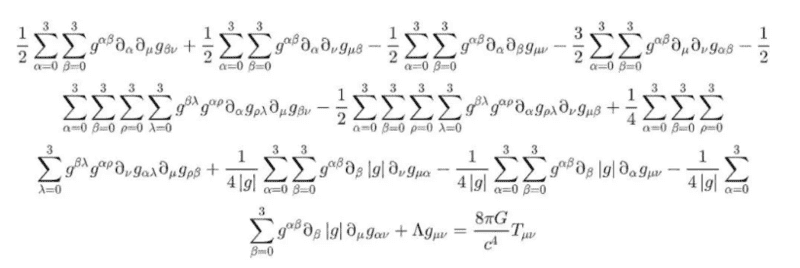from [here].

So what are the standard methods to solve (in 1 go) 16 differential equations in 10 variables?

If this is just computing power, solving for 2 or 3 or arbitrary body metrics should be routine, shouldn't it? What's the major obstacle that makes this very hard?

I know ADM probably does this, but many research level topics are couched in jargon that makes things appear harder than it is. A straightforward worked example is much preferable to get going.

Mentor
we would get 16 equations with 10 variables
No, we would get ten equations, one for each independent pair of indexes ##i## and ##j## (there are only 10 because the tensors involved are all symmetric, so 6 of the 16 total components are not independent, meaning 6 of the 16 total equations are duplicates of one of the others).

what are the standard methods to solve
The only method that works in the general case is numerical simulation.

If you know that the spacetime in question has particular symmetries, you can use those to simplify your ansatz for the metric, which in turn reduces the number of equations you have to solve (since with symmetries present some of the equations either duplicate others or reduce to nothing). Sometimes, if you're lucky, the resulting system can be solved exactly. For a very simple example with a high degree of symmetry, see this Insights article:

https://www.physicsforums.com/insig...-in-a-static-spherically-symmetric-spacetime/

•James1238765 and vanhees71
James1238765
thank you. I think I roughly understand the general analytic strategy now, ie. try to guess the functional form of the independent ##g_{ij}## components and their dependencies on ##\{t, r, \theta, \phi \}##, such that as many of ##\frac{dg_{ij}}{d?}## becomes zero, which causes many ##\Gamma## to become zero, which then causes many ##R^i_{jk}, R_{ij}## to become zero. The differential equations left over from the zeros might only have a few terms left, which may be amenable to analytic solving?

-------------------------------------

Playing the ##g_{ij}## guessing game and checking the resulting ##T_{ij}##, we notice:

1. Many arbitrary ##g_{ij}## gets disqualified because the matrix inverse ##g^{ij}## cannot be calculated at some point due to zero determinant.

2. Interesting hollow sphere type shapes can be obtained by different modifications, eg:

$$g_{ij} = \begin{bmatrix} \frac{1}{r}-\sin\theta & 0 & 0 & 0 \\ 0 & \frac{1}{1 - \frac{1}{r}} & 0 & 0 \\ 0 & 0 & r^2 & 0 \\ 0 & 0 & 0 & r^2\sin^2\theta \end{bmatrix}$$$$g_{ij} = \begin{bmatrix} \frac{1}{r}-1 & 0 & 0 & 0 \\ 0 & \frac{1}{1 - \frac{1}{r}} & 0 & 0 \\ 0 & 0 & r^2 & 0 \\ 0 & 0 & 0 & r^2\sin^2\theta\cos\phi \end{bmatrix}$$3. Due to the choice of spherical coordinates, any way of manipulating ##g_{ij}## will always give rise to sphere-type shapes (left-right symmetric). A metric that describes 2 bodies likely cannot be described in terms of ##g_{ij}(t,r,\theta,\phi)##, so we should better shift right away to a different coordinate system for ##g_{ij}##?Last edited:
Mentor
try to guess
No, you don't guess. You use whatever you know about the actual physical configuration you are trying to model. For example, if you know the spacetime is spherically symmetric, you can derive from that assumption a very simple form for the metric, as I did in the Insights article I referenced in an earlier post.

•vanhees71
2022 Award
thank you. I think I roughly understand the general analytic strategy now, ie. try to guess the functional form of the independent ##g_{ij}## components and their dependencies on ##\{t, r, \theta, \phi \}##, such that as many of ##\frac{dg_{ij}}{d?}## becomes zero, which causes many ##\Gamma## to become zero, which then causes many ##R^i_{jk}, R_{ij}## to become zero. The differential equations left over from the zeros might only have a few terms left, which may be amenable to analytic solving?
No. The approach is to think about your spacetime. Are there any differential symmetries? For example, if your spacetime is unchanging in some sense (like Schwarzschild spacetime) then there exists a coordinate system in which "space" is defined independently of time. That means that all the ##g_{0i}=g_{i0}=0## except ##g_{00}##. Furthermore, in this coordinate system the metric components must be independent of time. This simplifies your equations towards something tractable.

A two body problem does not have rotational symmetry. It does not have translational symmetry. It does not have time translation symmetry because orbiting bodies emit gravitational radiation and their orbits decay. The most you might have is a discrete symmetry or two and they don't help. So you can't simplify your metric by observation, and you have ten independent non-linear differential equations to solve simultaneously. Which is why numerical approximation is the tool for anything remotely realistic.

Last edited:
•vanhees71
romsofia
You should get this book:
https://www.amazon.com/dp/1108928250/?tag=pfamazon01-20

and eventually: https://www.amazon.com/dp/052151407X/?tag=pfamazon01-20

and lastly, a paper that you might find interesting: https://arxiv.org/pdf/1805.00813.pdf

They also have some code on https://www.cambridge.org/us/academ...lativity-starting-scratch?format=PB#resources if you click on the resources tab on the left, you can see the codes they use for chapters corresponding to the 1st book. Another tool is EinsteinToolKit.

You would benefit a lot more from going through a standard GR book first, but if you're dying to get started, then the 1st book is your best bet. As others have said, this problem isn't easy. And without knowing the actual background in GR, you don't even know what to expect. Will you ever truly know if your simulation is correct? What does the theory actually expect the answer to be? These are things that matter IMO.

•James1238765, vanhees71 and Ibix
James1238765
@romsofia thank you, the two books seem nicely computational! I have looked at a few books/articles on 3+1 method, a lot of new structures (folliation, ##\gamma##) seem needed for ADM & harmonic differential equations solving... I would like to try and exhaust simpler methods while reading up on standard methods. There are gradient-free optimization methods that might be suitable for these kinds of messy 10-component vector field input into a desired configuration of mass\vacuum output:

1. Genetic algorithm
2. Particle swarm
3. Simulated annealing

Have any of these been tried and shown to fail for solving the approximate 10 variables ##g_{ij}## distribution of numbers, given a desired output mass/vacuum configuration?

Fyi: I don't really wish for any particularly fast or efficient method for now, as I just wish to solve for ##g_{ij}## that leads to the correct ##T_{ij}## distribution. Many numerical methods have been optimized and developed for many decades, and really complicated methods exist for solving even basic differential equations. My wish is to pick the simplest (brute-force like if necessary) method that gives the right answer first. Slow or inefficient algorithms are not the main concerns for now, as the grid is really quite small 30x30x30x30.

Last edited:
James1238765
1. Random numerical ##g_{ij}## will generate valid ##T_{ij}##, with matter everywhere:Thus, the difficulty is in generating the black vacuum (zeros), instead of the (white) matter.

2. Combinatorially, we consider a 2x2x2 universe grid, with only 2 matter states (matter ON, and matter OF):The major difficulty is in generating any (black) vacuum at all in any subsquare. But supposing we have found a solution that turns off any subsquare:By symmetry of the cartesian dimensional axis, we can deduce the correct ##g_{ij}## codes to turn off any other single square configuration on the grid. Thus, knowing just 1 single-black-square solution can generate all other single-black-square solutions.

Similarly, know 2 distinct solutions that turns off 2 areas of the grid:andallows us by symmetry to deduce the ##g_{ij}## numbers that will turn off any 2-black-squares on the grid.

Further, knowing 2 distinct solutions that turns off 3 areas of the grid:andenables us by symmetry to deduce the ##g_{ij}## numbers that will turn off any 3-black-squares on the grid.

Then, knowing 4 distinct solutions that turns off 4 areas of the grid:(file limit reached)

allows us by symmetry to turn off any 4-black-squares on the grid.

There are 2 distinct solutions for 5-black-subsquares (ie. 3-white-subsquares).
There are 2 distinct solutions for 6-black-subsquares (ie. 2-white-subsquares).
There is 1 distinct solution for 7-black-subsquares (ie. 1-white-subsquares).
There is 1 distinct trivial solution for 8-black-subsquares (zero ##g_{ij}##).

Not counting the trivial solutions for all-white and all-black configurations, there are ##2+2+4+2+2=10## configuration solutions that must be discovered before we are masters of this universe, ie. are able to generate any of the ##2^8=256## possible matter states, and to evolve any sequence of matter states on this grid, eg:3. The unfortunate case of the Kerr solution is that it generates 1 single-white-square at the center of a 3x3 grid, for which no other solution can be deduced by symmetry.

Mentor
1. Random numerical ##g_{ij}## will generate valid ##T_{ij}##, with matter everywhere
In the sense that you can write down any metric you like, compute its Einstein tensor, divide by ##8 \pi##, and call that the "stress-energy tensor", yes. But whether the resulting SET describes anything that could physically exist is a very different question.

the difficulty is in generating the black vacuum (zeros), instead of the (white) matter.
On the contrary, solving the EFE for the case of vacuum is considerably easier.

The rest of your post is just handwaving and is getting considerably more off topic for this forum, since personal speculations are off limits.

Mentor
The OP question has been answered as well as it can be. Thread closed.